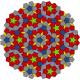# The monoclinic crystal system and the skew angle beta

One of the things we have tried to teach in our course is:

It is not the metric that determines to which crystal system a crystal belong. It is the other way round – the symmtry of the crystal determines the metric, although not in a biunique way.

In many textbooks the metric for the monoclinic crystal system is given as:

a ≠ b ≠ c, and αγ = 90°, β ≠ 90°  ;

And this is simply wrong. The correct statement is that there are no restrictions concerning a, b, and c, and there is also no restriction regarding the angle β, hence:

αγ = 90° – that’s it.

The important message here is that it is indeed not very likely that beta equals 90°, but it is not (mathematically) forbidden, the angle β could ‘accidentally’ be 90°.

While it is one thing to show mathematically that in the monoclinic crystal symmetry the maximum symmetry is 2/m and that therefore the angle beta can also be 90° it is another question if nature produces indeed a crystal belonging to the monoclinic crystal system and nonetheless possesses an angle β of 90°. This could then be considered as a sort of empirical proof.

Here it is 🙂

# Crystal structure of sodium potassium zinc diphosphate NaKZnP2O7

Yu.F. Shepelev, A.E. Lapshin, M.A. Petrova

Journal of Structural Chemistry

November 2006, Volume 47, Issue 6,

Space group P21/n, a = 12.585(5) Å, b = 7.277(5) Å, c = 7.428(5) Å, β = 90.00(5)° (!)

And this is how it looks like:PO4 tetrahedra (purple)
ZnO4 tetrahedra (grey)
sodium (yellow)
pottassium (blue)

## 5 thoughts on “The monoclinic crystal system and the skew angle beta”

1.Michael Fischer

You are completely right to point this out. But if you go this far about the angle beta, you should also make clear that pairs of a, b, and c (or all the three together) could be “accidentally” equal. Of course, the same is true in other crystal systems. In this sense, one would need a mathematical symbol that specifies “usually unequal, but can be accidentally equal”.

2.doktorholz Post author

Thanks Michael for your comment and this good idea – may be we can create such a sign by combining the “approx. equal” with the “unequal” sign?

best regards
Frank

3.Michael Fischer

Just to follow this up, I tried to find an extreme example: The mineral Villamananite has a pyrite-type structure, but the symmetry is reduced from cubic to monoclinic (space group P2_1). The lattice parameters still reflect the pseudo-cubic symmetry:
a = 5.709 b = 5.707 c = 5.708
beta= 90.01

(References and structure data can be found here: http://rruff.geo.arizona.edu/AMS/minerals/Villamaninite)